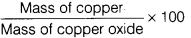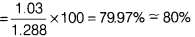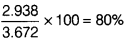# In which experiment, higher percentage of copper will be obtained? Show that these data verify the law of constant proportions

Two students performed the same experiment separately. In experiment I, 1.288 g of copper oxide is obtained from 1.03 g of copper. In experiment II, 3.672 g of copper oxide is obtained on oxidation of 2.938 g of copper. In which experiment, higher percentage of copper will be obtained? Show that these data verify the law of constant proportions.

Experiment I
Mass of copper oxide = 1.288 g
Mass of copper = 1.03 g
Percentage of copper =Percentage of oxygen = 100 - 80 = 20%
Experiment II
Mass of copper oxide = 3.672 g
Mass of copper = 2.938 g
Percentage of copper =Percentage of oxygen = 100 - 80 = 20%
In both the oxides, percentage compositions of copper and oxygen are 80% and 20% respectively. Hence, the law of constant proportions (law of definite composition) is verified.